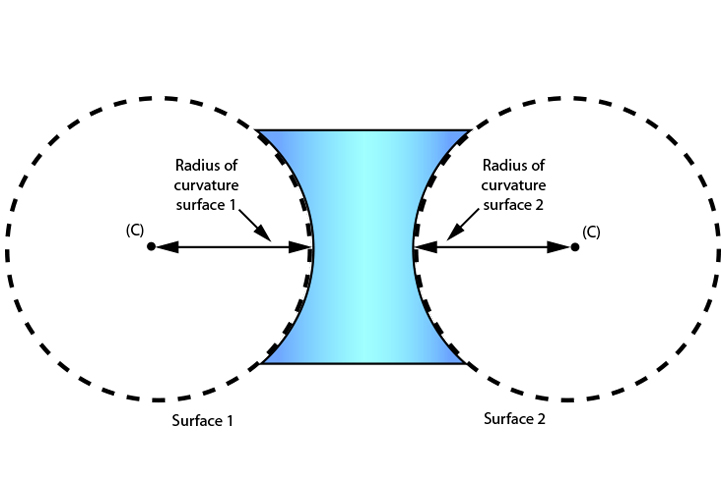# Concave lens – radius of curvature (R)

We should all know what a radius of a sphere is, so it should be easy to remember that:

The "radius of curvature" of a concave lens is the distance between the centre of curvature (C) and the curvature of sphere.

There are two radii of curvature for a concave lens, as below.